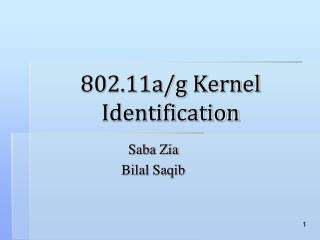DownloadDownload Presentation802.11a/g Kernel Identification

# 802.11a/g Kernel Identification

Télécharger la présentation## 802.11a/g Kernel Identification

- - - - - - - - - - - - - - - - - - - - - - - - - - - E N D - - - - - - - - - - - - - - - - - - - - - - - - - - -
##### Presentation Transcript

1. 802.11a/g Kernel Identification Saba Zia BilalSaqib

2. Physical Layer Architecture and Kernel Identification (802.11a) Ref: IEEE Std 802.11a-1999(R2003)

3. Physical Layer Architecture and Kernel Identification Data Scrambler/ Descrambler Convolutional Encoder / Viterbi decoder Data interleaver/ Deinterleaver Guard interval insertion OFDM modulation Subcarrier Modulation Mapping

4. Individual Properties of each KernelData Scrambler/Descrambler 127 bit frame synchronous scrambler S(x)=x7 +x4 +1 Ref: IEEE Std 802.11a-1999(R2003)

5. Individual Properties of each KernelConvolutional Encoder/ Viterbi Decoder • R = ½, 2/3, ¾ • For R= 1/2, G0=1338 G1 = 1718 • Decoding by Viterbi Algorithm Ref: IEEE Std 802.11a-1999(R2003)

6. Individual Properties of each KernelPuncturing/De-puncturing Patterns Ref: IEEE Std 802.11a-1999(R2003)

7. Individual Properties of each KernelPuncturing/De-puncturing Patterns Ref: IEEE Std 802.11a-1999(R2003)

8. Individual Properties of each KernelData Interleaver/ Deinterleaver • Block size corresponding to the number of bits in a single OFDM symbol, NCBPS • Two-step permutation • i = (NCBPS/16) (k mod 16) + floor(k/16) where k = 0,1,…,NCBPS – 1 • j = s × floor(i/s) + (i + NCBPS – floor(16 × i/NCBPS)) mod s where i = 0,1,… NCBPS – 1 • The value of s is determined by the number of coded bits per subcarrier, NBPSC, according to s = max(NBPSC/2,1)

9. Individual Properties of each KernelSubcarrier Modulation Mapping BPSK,QPSK,16 QAM or 64 QAM depending on the rate requested Gray coded constellation mappings Resultant, d = (I + jQ) X KMOD Ref: IEEE Std 802.11a-1999(R2003)

10. Individual Properties of each KernelOFDM modulation (IFFT) Divide the complex number string into groups of 48 complex numbers. Each such group will be associated with one OFDM symbol. Each complex number is mapped into OFDM subcarriers numbered –26 to –22, –20 to –8, –6 to –1, 1 to 6, 8 to 20, and 22 to 26. The “0” subcarrier, associated with center frequency, is omitted and filled with zero value. Four subcarriers are inserted as pilots into positions –21, –7, 7, and 21. The total number of the subcarriers is 52 (48 + 4). For each group of subcarriers –26 to 26, convert the subcarriers to time domain using inverse Fourier transform

11. Individual Properties of each KernelOFDM modulation (IFFT) Ref: IEEE Std 802.11a-1999(R2003)

12. Individual Properties of each KernelGuard Interval Insertion Prepend to the Fourier-transformed waveform a circular extension of itself thus forming a GI, and truncate the resulting periodic waveform to a single OFDM symbol length by applying time domain windowing. Ref: IEEE Std 802.11a-1999(R2003)

13. Rate Dependent Parameters Ref: IEEE Std 802.11a-1999(R2003)

14. Timing Related Parameters Ref: IEEE Std 802.11a-1999(R2003)

15. Physical Layer Architecture and Kernel Identification (802.11g) • 802.11g offers four operational modes • DSSS /CCK • OFDM (Kernels identical to 802.11a) • PBCC (optional) • DSSS/OFDM (optional)

16. DSSS/CCK based kernels CRC Data Scrambler/Descrambler DQPSK/DBPSK